# 【记录】Excel中的时间的特殊性导致TIME值变成介于0到1之间的值，不是我们想要看到的h:mm的形式

Excel 1719浏览

【问题】

【记录】Excel内置函数实现两列的时间相减以及条件格式化

8:31-6.00=2小时31分钟=2小时，31/60=0.517=2.517小时

`=TIMEVALUE(TEXT(\$H718,"h:mm")) - TIME(VALUE(MID(\$J718,1,1)),VALUE(MID(\$J718,3,2)),0)`

0.105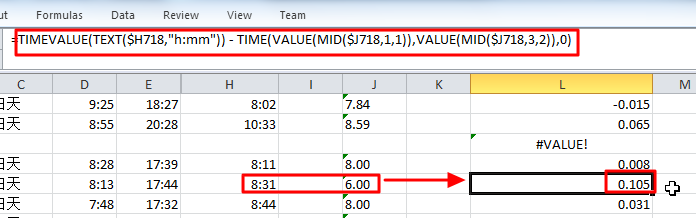【折腾过程】

1.后来注意到，6.00是x.xx其中xx是小数，而不是直接的分钟值，所以去修正公式为：

`=TIMEVALUE(TEXT(\$H718,"h:mm")) - TIME(VALUE(MID(\$J718,1,1)),VALUE(MID(\$J718,3,2))*60/100,0)`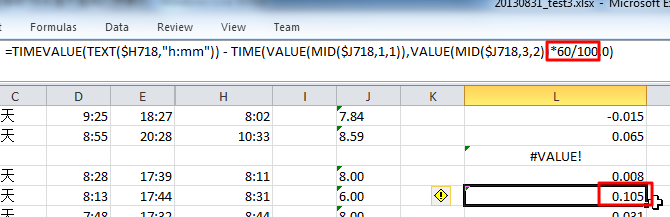（后来注意到，因为此处6.00中小数部分是00，所以结果00*60/100，肯定还是00，的确是应该没变化的）

2.后来经过折腾发现：

`=MID(\$H718,1,4)`

8:31

0.35

（之前是：

`=MID(\$H718,1,3)`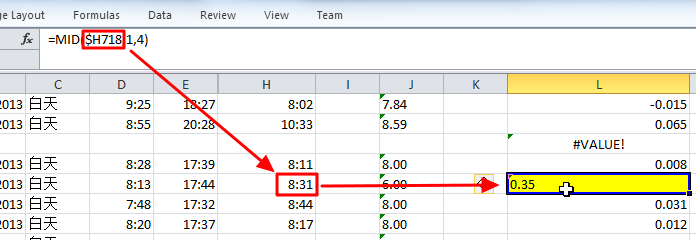3.所以，看出此处的值，有些异常。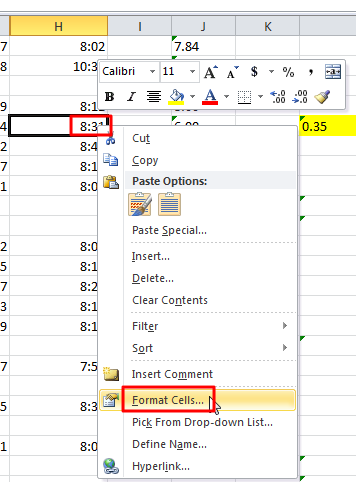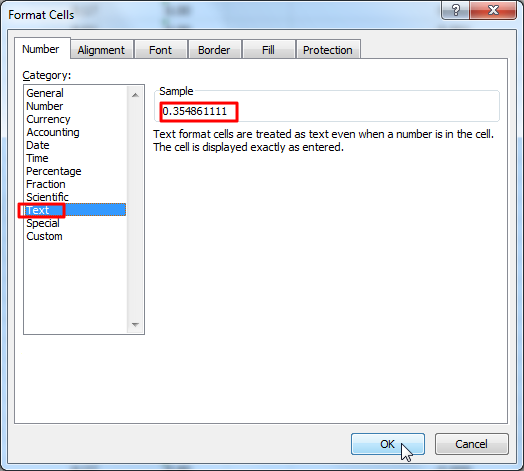0.354861111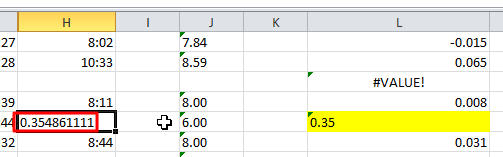h:mm

4.对此，很是迷惑。没搞懂。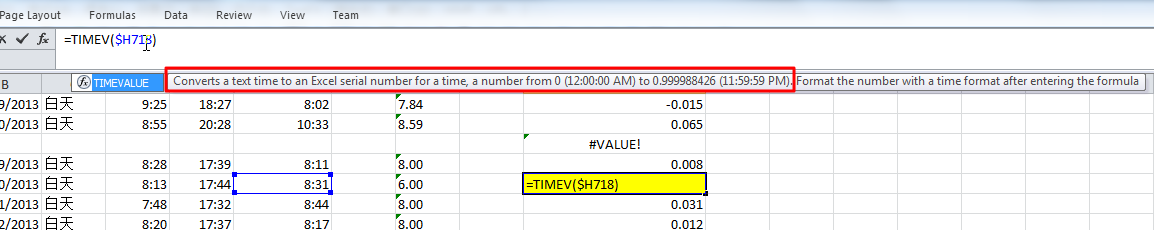Convert a text time to an Excel serial number for a time,a number from 0(12:00:00 AM) to 0.999988426（11:59:59PM).Format the number with a time format after entering the formula.

8:31

 =TIMEVALUE(TEXT(\$H718,"h:mm")) – TIME(VALUE(MID(\$J718,1,1)),VALUE(MID(\$J718,3,2))*60/100,0)

 TIMEVALUE(TEXT(\$H718,"h:mm"))

 =\$H718 – TIME(VALUE(MID(\$J718,1,1)),VALUE(MID(\$J718,3,2))*60/100,0)

0.105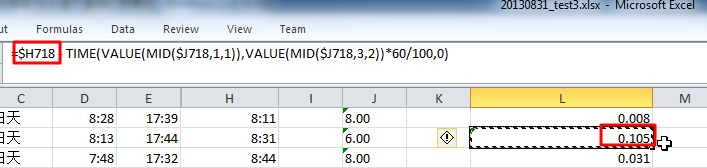5.然后自己突然想起来，估计乘于24，就是所需要的值了。

 =(\$H718 – TIME(VALUE(MID(\$J718,1,1)),VALUE(MID(\$J718,3,2))*60/100,0))*24

2.517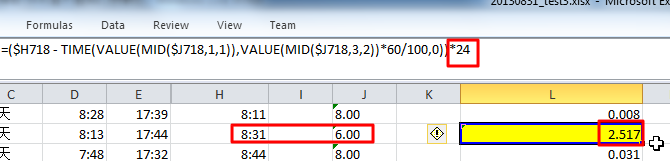【总结】

excel中的TIME所得到的值，不是我们所希望的，小时，分钟，时间的，而直接表示出来的值

2.517小时

2.517小时/24小时=0.104875=0.105了。

98 queries in 0.220 seconds, using 23.10MB memory